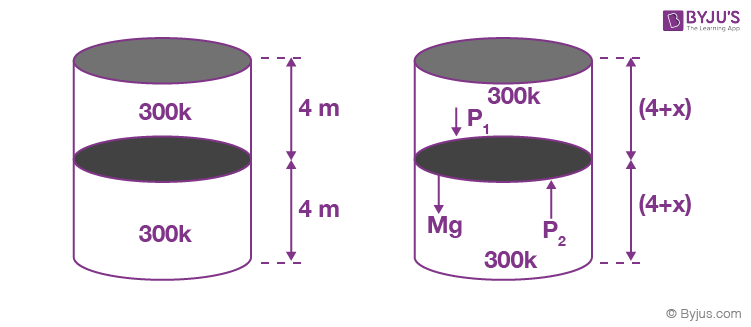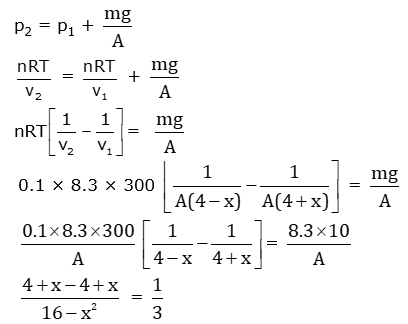# A thermally isolated cylindrical closed vessel of height 8 m is kept vertically. It is divided into two equal parts by a diathermic (perfect thermal conductor) frictionless partition of mass 8.3 kg. Thus the partition is held initially at a distance of 4 m from the top, as shown in the schematic figure below. Each of the two parts of the vessel contains 0.1 mole of an ideal gas at temperature 300 K. The partition is now released and moves without any gas leaking from one part of the vessel to the other. When equilibrium is reached, the distance of the partition from the top (in m) will be _______

(take the acceleration due to gravity = 10 ms−2 and the universal gas constant = 8.3 J mol−1K−1).6x = 16 – x2

x2 + 6x – 16 = 0

x = –8, 2

The distance of the partition from the top = 4 + 2 = 6 m(1)(2)